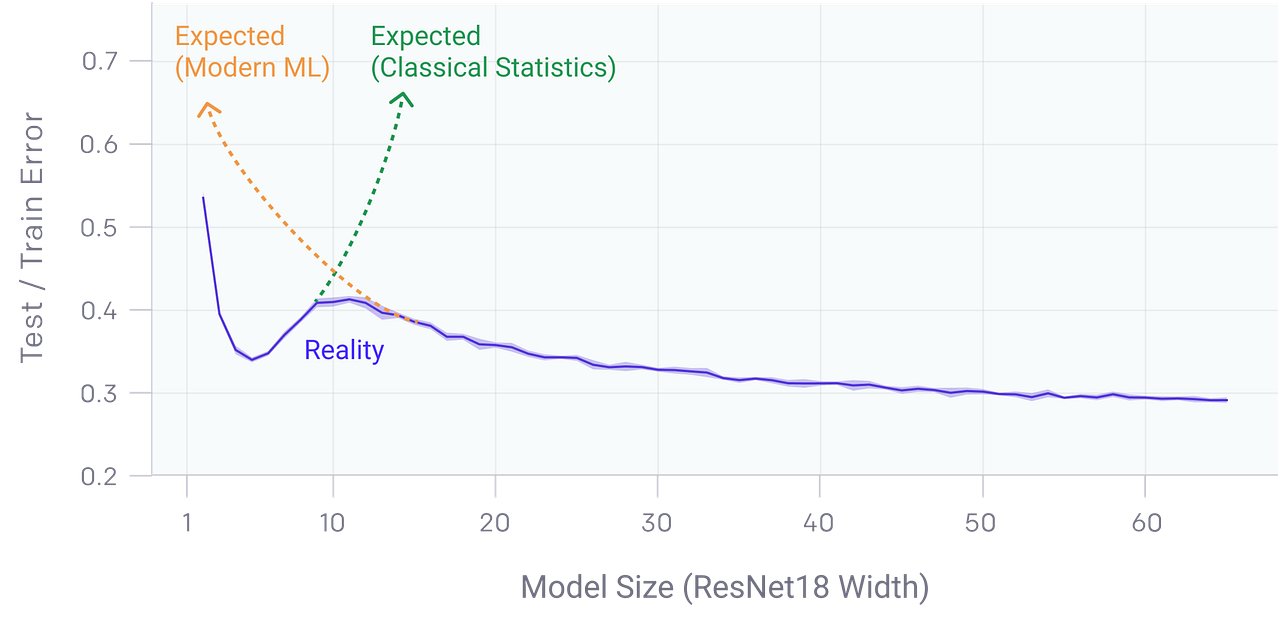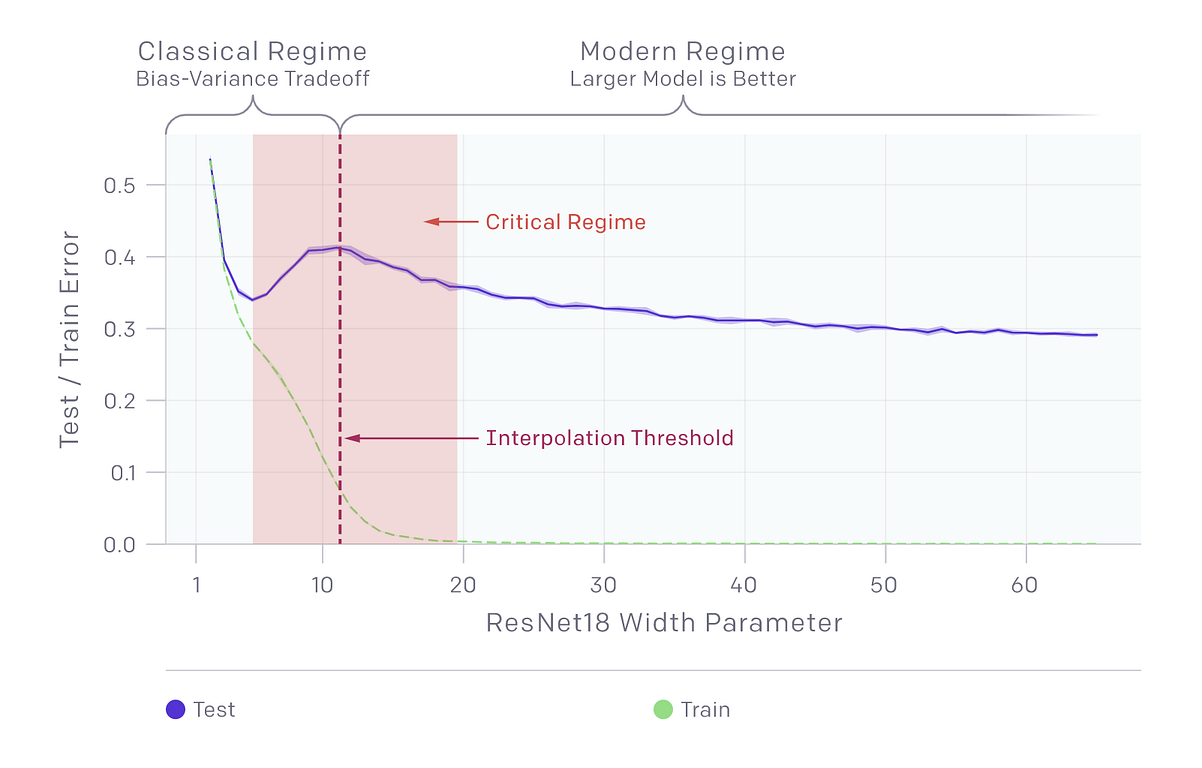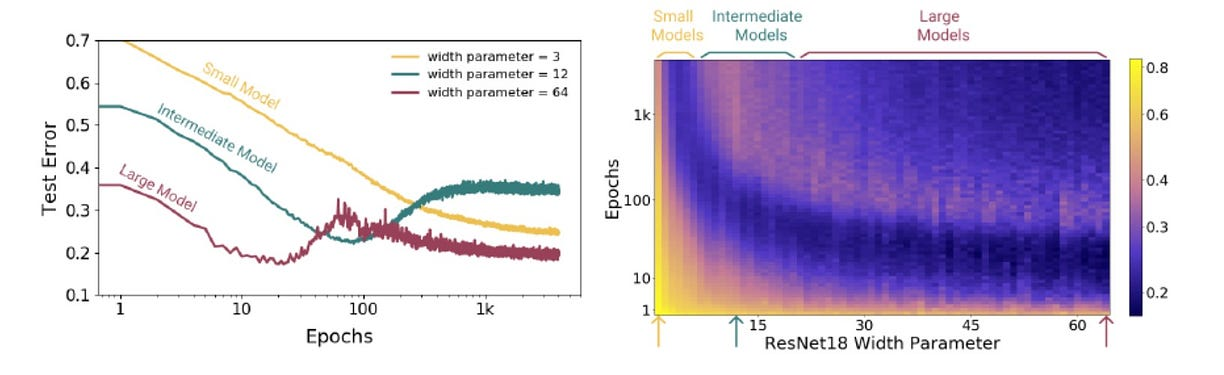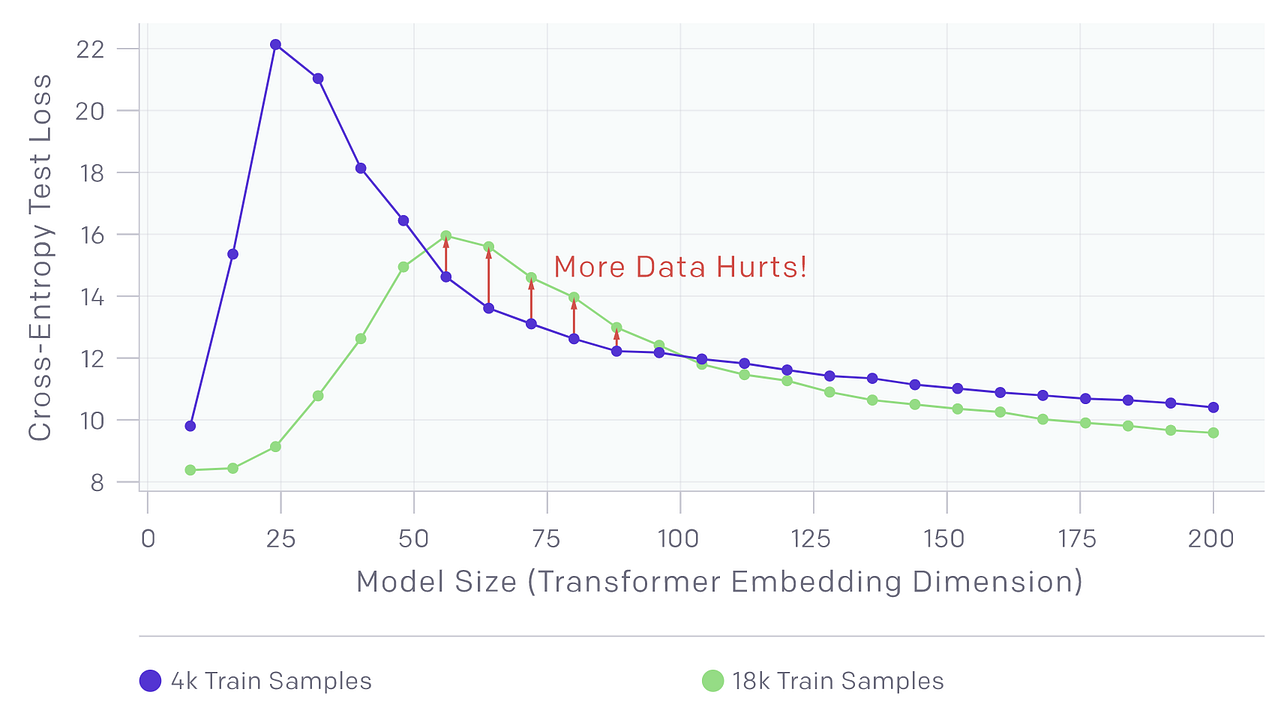# The Double Descent Hypothesis: How Bigger Models and More Data Can Hurt Performance

OpenAI research shows a phenomenon that challenges both traditional statistical learning theory and conventional wisdom in machine learning practitioners.Bigger is better certainly applies to modern deep learning paradigms. Large neural networks with millions of parameters have regularly outperformed collections of smaller networks specialized on a given task. Some of the most famous models of the last few years such as Google BERT, Microsoft T-NLG or OpenAI GPT-2 are so large that their computational cost results prohibited for most organizations. However, the performance of a model does not increase linearly with its size. Double descent is a phenomenon where, as we increase model size, performance first gets worse and then gets better. Recently, OpenAI researchers studied how many modern deep learning models are vulnerable to the double-descent phenomenon.

The relationship between the performance of a model and its size have certainly puzzled deep learning researchers for years. In traditional statistical learning, the bias-variance trade off states that models of higher complexity have lower bias but higher variance. According to this theory, once model complexity passes a certain threshold, models “overfit” with the variance term dominating the test error, and hence from this point onward, increasing model complexity will only decrease performance. From that perspective, statistical learning tells us that “larger models are worse”. However, modern deep learning model have challenged this conventional wisdom.

Contrasting with the bias-variance tradeoff, deep neural networks with millions of parameters have proven to outperform smaller models. Additionally, many of these models improve linearly with more training data. Therefore, the conventional wisdom among deep learning practitioners is that “larger models and more data are always better”.

Which theory is correct? Statistical learning or the empirical evidence of deep learning models? The double descent phenomenon show us that both theories can be reconciled but, also, that some of its assumptions are erroneous.

### Deep Double Descent

An easy way to think about the double descent phenomenon is that

“things get worse before they get better when training a deep learning model”.

In many traditional deep learning models such as CNNs, RNNs or transformers, we can notice that the performance peak occurs predictably at a “critical regime,” where the models are barely able to fit the training set. As the number of parameters in the neural network increases, the test error initially decreases, increases, and, just as the model is able to fit the train set, undergoes a second descent.

The main point of the deep double descent theory is that neither classical statisticians’ conventional wisdom that “too large models are worse” nor the modern deep learning paradigm that “bigger models are better” uphold. It all depends of the state of the model. OpenAI outlines this concept in a very simple mathematical model known as the double descent hypothesis.

### Double Descent Hypothesis

Let’s introduce the notion of effective model complexity(EMC) for a training procedure T. The EMC of T or EMC(T) would be the maximum number of samples n on which T achieves on average approximately 0 training error. Using that definition, we can classify a deep learning model in three critical states:

• Under-Parameterized: If EMC(T) is sufficiently smaller than n, any perturbation of T that increases its effective complexity will decrease the test error.
• Over-Parameterized: If EMCD(T ) is sufficiently larger than n, any perturbation of T that increases its effective complexity will decrease the test error.
• Critically-Parameterized: If EMCD(T) ≈ n, then a perturbation of T that increases its effective complexity might decrease or increase the test error

By qualifying the state of the model, the deep double decent hypothesis sheds light on the interaction between optimization algorithms, model size, and test performance and helps reconcile some of the competing intuitions about them. In the under-parameterized regime, where the model complexity is small compared to the number of samples, the test error as a function of model complexity follows the U-like behavior predicted by the classical bias/variance tradeoff. However, once model complexity is sufficiently large to interpolate i.e., achieve (close to) zero training error, then increasing complexity only decreases test error, following the modern intuition of “bigger models are better”.

Following the deep double descent hypothesis, OpenAI observed three key states during the learning lifecycle of a model.

1) Model-Wise Double Descent: Describes a state where bigger models are worse.

2) Epoch-Wise Double Descent: Describes a state where training longer reverses overfitting.

3) Sample-Wise Non-Monotonicity: Describes a state where more samples hurts the performance of the model.

### Model-Wise Double Descent

The model-wise double descent describe a phenomenon when the model is under-parameterized. In that state, the peak in test error occurs around the interpolation threshold, when the models are just barely large enough to fit the train set. The model-wise double descent phenomenon also shows that changes which affect the interpolation threshold (such as changing the optimization algorithm, the number of train samples, or the amount of label noise) also affect the location of the test error peak correspondingly.### Epoch-Wise Double Descent

The epoch-wise double descent describes a state where sufficiently large model transitions from under- to over-parameterized over the course of training. In that state, sufficiently large models can undergo a “double descent” behavior where test error first decreases then increases near the interpolation threshold, and then decreases again. In contrast, for “medium sized” models, for which training to completion will only barely reach a level of error close to 0, the test error as a function of training time will follow a classical U-like curve where it is better to stop early. Models that are too small to reach the approximation threshold will remain in the “under parameterized” regime where increasing train time monotonically decreases test error.### Sample-Wise Non-Monotonicity

The sample-wise non-monotonicity refers to a state in which adding more training samples hurts the performance of the model. Specifically, increasing the number of samples has two different effects on the test error vs. model complexity graph. On the one hand, (as expected) increasing the number of samples shrinks the area under the curve. On the other hand, increasing the number of samples also has the effect of “shifting the curve to the right” and increasing the model complexity at which test error peaks.The double descent hypothesis adds some interesting context to helps understand the performance of deep learning model over time. The practical experiments show that neither the statistical learning theory that neither classical statisticians’ conventional wisdom that “too large models are worse” nor the modern deep learning paradigm that “bigger models are better” are entirely correct. Factoring in the deep double descent phenomenon can be an important tool for deep learning practitioners as it emphasizes on the importance of choosing the right dataset, architecture, and training procedures to optimize the performance of a model.

Original. Reposted with permission.

Related: